# Scope

## State of the art

The numerical treatment of parameter identification problems for partial differential equations (PDEs) arising in diverse areas of science and (industrial or economic) applications has received an increasing amount of attention in the recent past. In many situations these problems can be formulated as optimal control problems for PDEs. For the optimization of systems governed by PDEs, second-order algorithms, such as Newton or sequential quadratic programming (SQP) methods have been developed and analyzed in detail. The augmented Lagrange-SQP method and its inexact variant - including globalization strategies - was analyzed by scientists of the University of Graz, for instance.

Parameter estimation of engineering components or systems often requires repeated, reliable, and real-time prediction of the parameters such as forces, critical stresses, flow rates or heat fluxes. In recent publications, efficient optimization methods such as the augmented Lagrange-SQP method have been combined with a model reduction based on proper orthogonal decomposition (POD). POD is a method for deriving low order models for systems of differential equations. It is based on projecting the system onto subspaces consisting of basis elements that contain characteristics of the expected solution. The POD basis in parabolic problems is derived from solutions to the underlying PDE for different time-instances. In applications where we face elliptic parameter-dependent systems, the POD basis is derived from solutions to the underlying PDE for different parameter values.

Generally, we will concentrate on problems to estimate parameters μ in a parameter space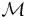m, m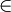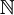, from measurements ud for either the solution u=u(μ), μ, to the elliptic variational problem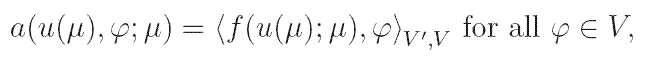where V is an appropriate Hilbert space with dual V', a( · , · ;μ): V ū Vdenotes a bilinear form depending on the parameter μand f( · ;μ): VV' is a non-linear mapping, which may depend also on the parameter μ, or for the solution u=u(t,μ), (t,μ)[0,T] ū, to the parabolic variational problem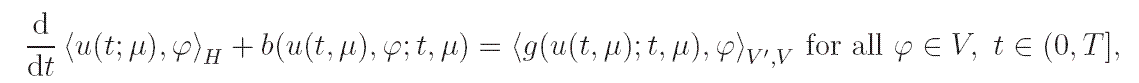where HV is a Hilbert space that is dense in V, b( · , · ;t,μ): V ū Vdenotes a bilinear form and g( · ;t,μ): VV' is a non-linear mapping both depending on the time t[0,T] and on the parameter μ.
In applications, the parameters, that have to be identified, typically satisfy certain inequality constraints. For instance, the parameters are bounded from below and above for physical or technical reasons. Hence, we suppose that the parameter setm has the form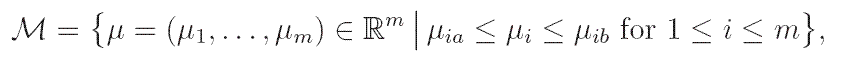where μia and μib denote lower and upper bounds, respectively, for the i-th component of the parameter μ.
The resulting parameter estimation problem can be written as a constrained optimal control problem.

## Aims of the project

The main focus of the project is the development of efficient reliable solution methods for constrained parameter estimation problems governed by a class of elliptic as well as parabolic non-linear PDEs, where the parameters are scalars or constants on subdomains.

The POD method is compared to other model reduction methods, such as reduced-basis element methods or balanced truncation, and we plan to use these methods within our algorithm.

We also aim to apply our technique on an example arising in (industrial) applications. In the development of modern vehicles efficient and precise prediction techniques are needed for the vehicle interior noise. The steady-state sound pressure p satisfies the Helmholtz equation, which depends non-linearly on the frequency parameter. While the sound pressure is measurable utilizing technical devices, the involved parameters can often not be measured directly. Therefore, parameter identification problems governed by the Helmholtz equation are important and interesting in (industrial) applications. We aim to study POD Galerkin approximations for the Helmholtz equation and utilize these approximations within this (non-linear) parameter estimation problem.

We will carry out error analysis for the discretized problems and discuss issues like choice of snapshots or topology for the POD basis computation. First steps are already done for scalar parameters. These results have to be extended to several parameters or for parameters which are constant on subdomains. Moreover, non-linear dependence on the parameters is an interesting issue. This is, e.g., the case for the Helmholtz equation.

Furthermore, a-posteriori analysis can be carried out for the POD solution by solving a additional adjoint problem. In a cooperation with Prof. F. Tröltzsch (Berlin University of Technology) we plan to incorporate a-posteriori POD error analysis in the POD solver.

As optimization methods we aim to use augmented Lagrange algorithms combined with globalized SQP or Newton methods or semi-smooth Newton variants. Since the augmented Lagrange SQP method has already been investigated in this context in previous papers, we will concentrate on semi-smooth Newton methods, which turn out to be very efficient for box constrained optimal control problems, for example. It was shown that the active set strategy is equivalent to a semi-smooth Newton method and, as a consequence, that it converges at a locally superlinear rate. Since Hessian information is utilized, the semi-smooth Newton method is a second-order method. Second-order information typically speeds up the convergence locally near a solution of the optimal control problem satisfying second-order sufficient optimality conditions. The designed algorithms are tested with respect to global convergence, rate of convergence and efficiency.

In the parameter estimation for hyperthermia problems on-line strategies are needed to control the individual medical treatment of a patient during an application. We aim to provide a POD discretization to on-line parameter estimation problems arising in hyperthermia that can be used for fast and reliable computations in a cooperation with Dr. Weiser from the Zuse Institute Berlin.

In our project we will also focus on time-dependent problems. Scalar parameters in the linear and non-linear heat equation are estimated. Here we aim to utilize the semi-smooth Newton method.

Moreover, we plan to extend our error analysis to parameter estimation problems that can be written as linear-quadratic optimal control problems. The work shall be also influenced by the cooperation with Prof. Dr. M. Hinze from the University of Hamburg.

The solution methods which we will develop in our project will be implemented and tested in MATLAB utilizing the FEMLAB package for the finite element discretization.